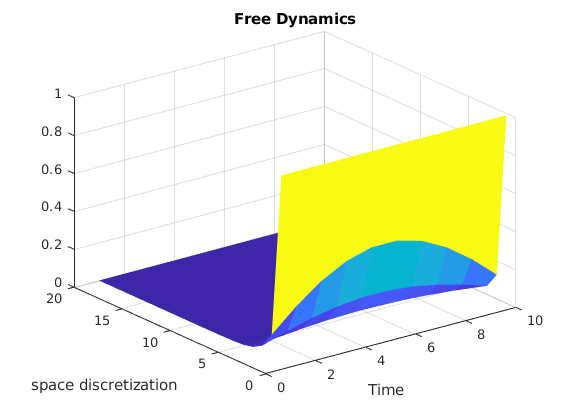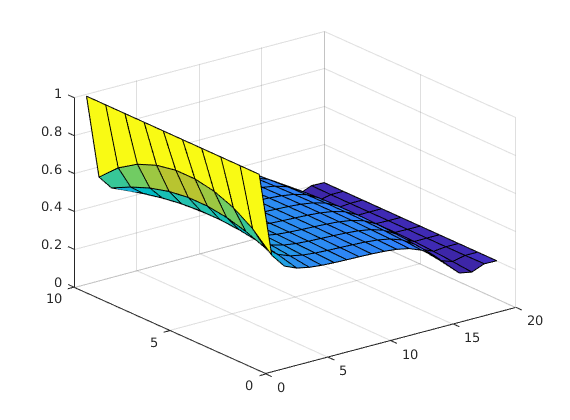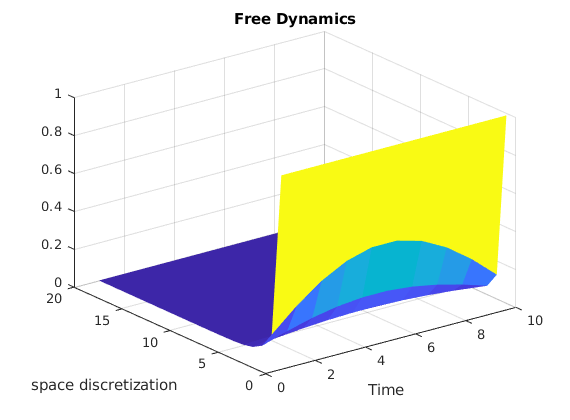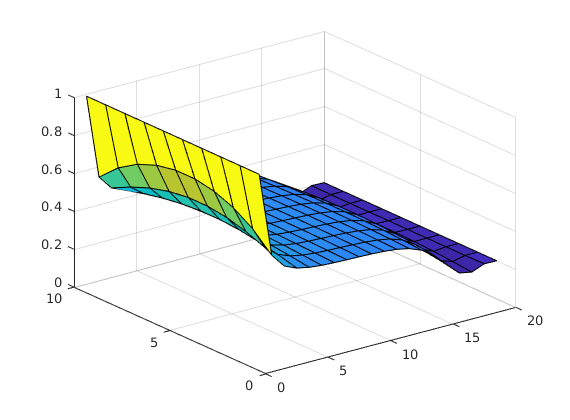# Population Dynamics

To start this tutorial, write the following command in the MATLAB console

open T0010_sl_constraints

## Semi-linear semi-discrete heat equation and collective behavior

Definition of the time

clear
syms t
%% Discretization of the space
N = 10;
L = 1;
xi = 0; xf = L;
xline = linspace(xi,xf,N+2);
xline = xline(2:end-1);

symY = SymsVector('y',N);
symU = SymsVector('u',1);


We create the functional that we want to minimize Our goal is to set the system to zero penalizing the norm of the control by a parameter $\beta$ that will be small.

YT = 0.2 + 0*xline';
dx = xline(2) - xline(1);
symPsi  = @(T,symY) (YT - symY).'*(YT - symY);
symL    = @(t,symY,symU) 0 ;


We create the ODE object Our ODE object will have the semi-discretization of the semilinear heat equation. We set also initial conditions, define the non linearity and the interaction of the control to the dynamics.

Initial condition

%%Y0 = 2*sin(pi*xline)';
Y0 = 0.99+0*xline';


Diffusion part: the discretization of the 1d Laplacian

A = FDLaplacian(xline);


We define the matrix B that will be the effect of the interior control to the dynamics

B =  (N^2/L^2)*[1 ; zeros(N-2,1) ;1];


Definition of the non-linearity $\partial_y[-5\exp(-y^2)]$

syms x G(x) U(x) DG(x);
%%
G(x)=x*(1-x)*(x-0.2);
formula=G(x);
G = symfun(formula,x);


and we define the part of the dynamics corresponding to the nonlinearity

vectorF = arrayfun( @(x)G(x),symY);


Putting all the things together

Fsym  = A*symY + vectorF + B*symU;
syms t
Fsym_fh = matlabFunction(Fsym,'Vars',{t,symY,symU,sym.empty});

odeEqn = pde(Fsym_fh,symY,symU,'InitialCondition',Y0,'FinalTime',2.0);
odeEqn.Nt=20;
odeEqn.mesh{1} = xline;
odeEqn.Solver = @ode23tb;


We solve the equation and we plot the free solution applying solve to odeEqn and we plot the free solution.

solve(odeEqn)


ans =

Columns 1 through 7

0    0.1053    0.2105    0.3158    0.4211    0.5263    0.6316

Columns 8 through 14

0.7368    0.8421    0.9474    1.0526    1.1579    1.2632    1.3684

Columns 15 through 20

1.4737    1.5789    1.6842    1.7895    1.8947    2.0000


figure;
surf(odeEqn.StateVector.Numeric,'EdgeColor','none');
title('Free Dynamics')
ylabel('space discretization')
xlabel('Time')We create the object that collects the formulation of an optimal control problem by means of the object that describes the dynamics odeEqn, the functional to minimize Jfun and the time horizon T

iCP1 = Pontryagin(odeEqn,symPsi,symL);
%%
iCP1.Constraints.MaxControl = 1;
iCP1.Constraints.MinControl = 0;

AMPLFile(iCP1,'Domenec.txt')
out = SendNeosServer('Domenec.txt');


Output in: /home/djoroya/Documentos/GitHub/DyCon-toolbox/tmp/AMPL-executions/26-Sep-2019-11-11-55627-776095-Domenec.txt/Domenec.txt.outThe control function inside the control region

figure;
surf(data.State)